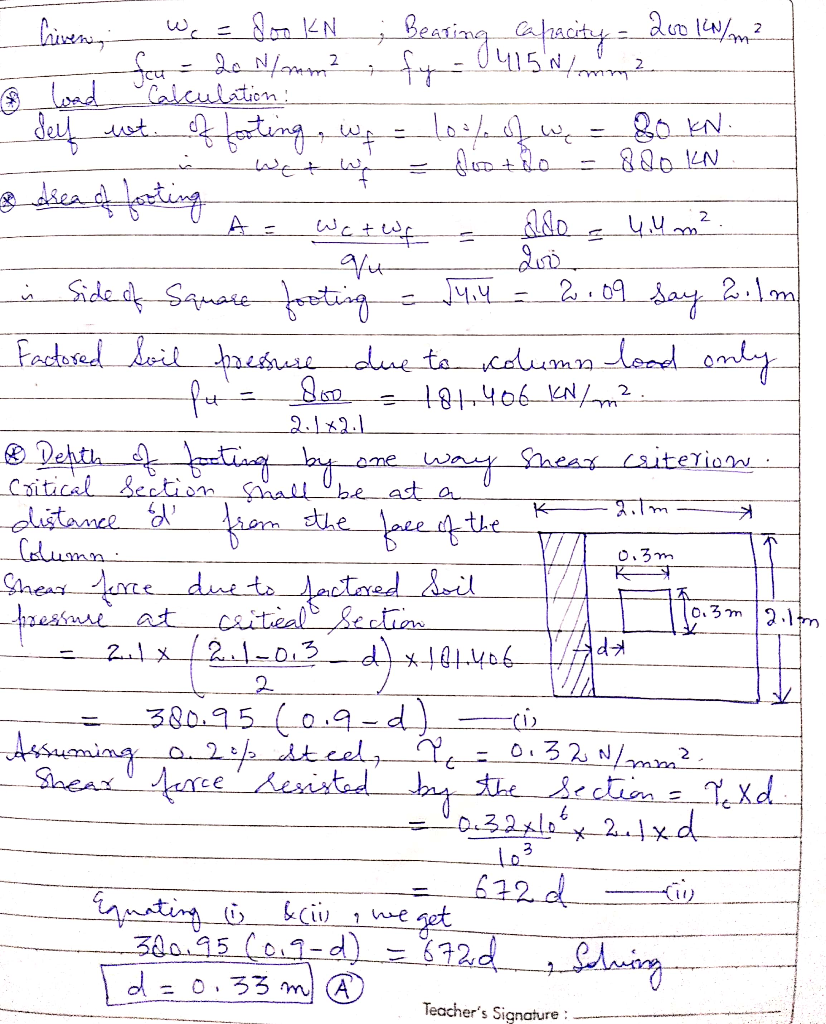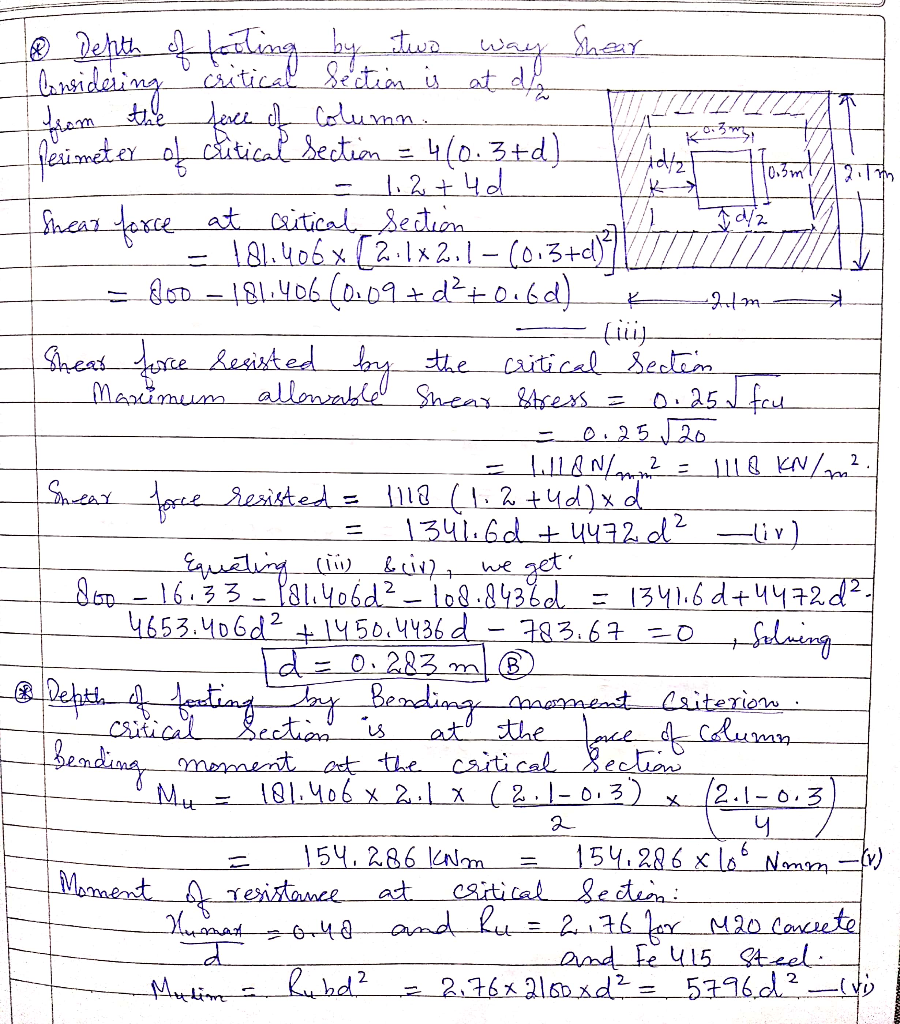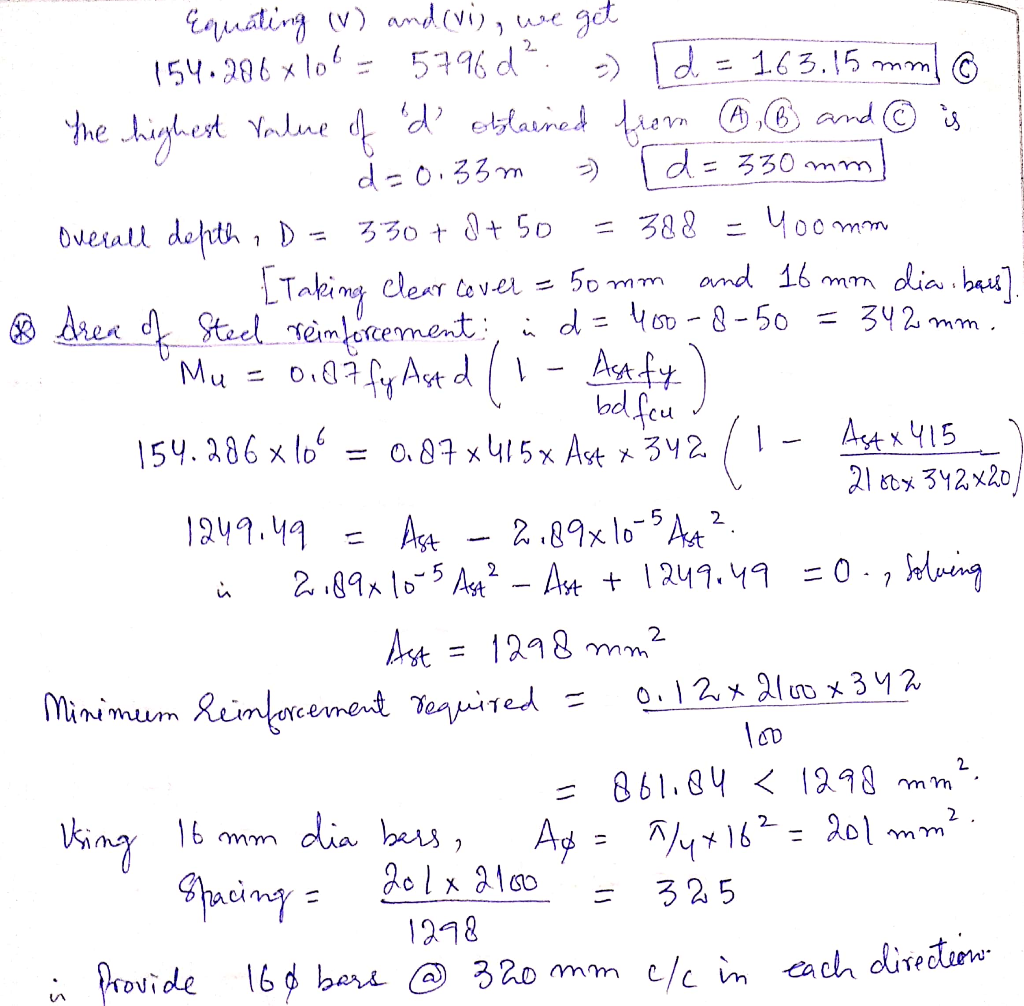##### Question

In: Civil Engineering

# Design for britishcode 8110 Design a square pad footing for a 300 mm square column supporting...

Design for britishcode 8110

Design a square pad footing for a 300 mm square column supporting a factored axial load of 800 kN. Safe bearing capacity of soil is 200 kN/m2, fcu=20 N/mm2, fy=415 N/mm2.Design a square pad footing for a 300 mm square column supporting a factored axial load of 800 kN. Safe bearing capacity of soil is 200 kN/m2, fcu=20 N/mm2, fy=415 N/mm2.

## Solutions

##### Expert Solution## Related Solutions

##### The detail of column and pad footing are as under:
The detail of column and pad footing are as under:                                                                                              Size of column: 300 mm x 300 mm.                                                                                            Live load on column: 900 kN.           Dead load on column: 1200 kN.           Soil Bearing Pressure: 180 kN/m² and Depth of footing as 400 mm           Nominal cover: 50 mm and 20 mm diameter bars for reinforcement Design the footing for bending.      Check the footing in two way shear.          iii. Write the reasons for...
##### QUESTIONS: Design a square column footing for a 20-in. square tied interior column that supports loads...
QUESTIONS: Design a square column footing for a 20-in. square tied interior column that supports loads of DL (170) k and Live load LL (210) k. The column is reinforced with eight No 8 bars, the bottom of the footing is 5 foot below final grade, and the soil weighs 100 lb. /ft3 the allowable soil pressure is 4 w ksf. The concrete strength is 4,000 psi and the steel is Grade 60.
##### . Design a square footing for a 16-in. square interior column with an ultimate dead load...
. Design a square footing for a 16-in. square interior column with an ultimate dead load = 300 (k) and an ultimate live load = 200 (k). Take fy=60,000 psi, fc=4000 psi, normal weight, and qa= 4500 psf. Assume the base of the footing is 4 (ft.) below grade. Draw the reinforcement detail also. Check for one way and two way shears with the support of diagram. With ACI code
##### Design a square footing for a 18-inch. square column and a superimposed dead load of 200...
Design a square footing for a 18-inch. square column and a superimposed dead load of 200 kips and a live load of 300 kips. The maximum permissible soil pressure is 4000 psf. Use concrete with a design strength of 3 ksi and grade 40 reinforcing bars with yield strength of 40 ksi.
##### Q.2. Design a square footing for a 16-in. square interior column with an ultimate dead load...
Q.2. Design a square footing for a 16-in. square interior column with an ultimate dead load = W1 (k) and an ultimate live load = W2 (k). Take fy=60,000 psi, fc=4000 psi, normal weight, and qa= (w) psf. Assume the base of the footing is h (ft.) below grade. Draw the reinforcement detail also. Check for one way and two way shears with the support of diagram.                                                                                                       Student shall take the data given below in the table against...
##### design for british code 8110 1.A 500 mm square column carries a dead load of 1200...
design for british code 8110 1.A 500 mm square column carries a dead load of 1200 kN and imposed load of 400 kN. The safe bearing capacity of the soil is 180 kN/m2. Design a square pad footing to resist the loads using the following material strengths: fcu=30 N/mm2, fy=500 N/mm2. 2.Design a pad footing for a rectangular column of section 300x500 mm supporting an axial factored load of 1,400 kN. The safe bearing capacity of the soil is 180...
##### Design an isolated rectangular footing to support an interior column 40 cm × 40 cm in...
Design an isolated rectangular footing to support an interior column 40 cm × 40 cm in cross section and carries a dead load of 800 kN and a live load of 600 kN. Use f́c= 21 MPa, fy= 420 MPa, q all (net) =200 kN / m2 , γsoil = 17 kN /m3 , and Df =1.25 m. Space limitations are such that one lateral dimension cannot exceed 2.5 m
##### Design an individual column footing where DL = 200k, LL = 500k, allowable soil pressure =...
Design an individual column footing where DL = 200k, LL = 500k, allowable soil pressure = 3k/ft2, f′c col = 4ksi, f′c ftg = 3ksi, 28″ x 28″ column, supporting interior column.
##### Design an individual column footing where DL = 200k, LL = 500k, allowable soil pressure =...
Design an individual column footing where DL = 200k, LL = 500k, allowable soil pressure = 3k/ft2, f′c col = 4ksi, f′c ftg = 3ksi, 28″ x 28″ column, supporting interior column.
##### Explain the way to construct pad footing step by step with sketch. 1) Setting Out 2)...
Explain the way to construct pad footing step by step with sketch. 1) Setting Out 2) Excavation Works 3) Install Formwork 4) Install Reinforcement 5) Inspection of workdone before concreting 6) Concreting work